Next: Arne Ledet - Some Up: Number Theory / Théorie Previous: Hershey Kisilevsky - Rank

## Manfred Kolster - Higher relative class number formulas

 MANFRED KOLSTER, Department of Mathematics, McMaster University, Hamilton, Ontario  L8S 4K1, Canada Higher relative class number formulas

Let F/F+ be a CM extension of number fields, and let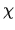denote the non-trivial character of the Galois group of F/F+. The classical relative class number formula gives the following relation between the value of the L-function ofat 0 and the relative class number h-: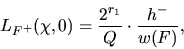where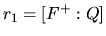, Q is the so-called Q-index, and w(F)is the number of roots of unity in F. The purpose of the talk is to describe analogs of this formula relating the values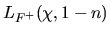for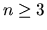an odd integer to the orders of relative motivic cohomology groups and K-groups.Next: Arne Ledet - Some Up: Number Theory / Théorie Previous: Hershey Kisilevsky - Rank